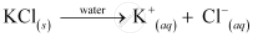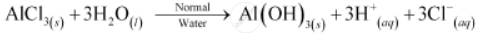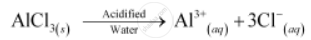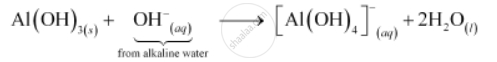Share

# Do You Expect Different Products in Solution When Aluminium (Iii) Chloride and Potassium Chloride Treated Separately with (I) Normal Water (Ii) Acidified Water, and (Iii) Alkaline Water? Write Equations Wherever Necessary. - Chemistry

ConceptWater Chemical Properties of Water

#### Question

Do you expect different products in solution when aluminium (III) chloride and potassium chloride treated separately with (i) normal water (ii) acidified water, and (iii) alkaline water? Write equations wherever necessary.

#### Solution

Potassium chloride (KCl) is the salt of a strong acid (HCl) and strong base (KOH). Hence, it is neutral in nature and does not undergo hydrolysis in normal water. It dissociates into ions as follows:In acidified and alkaline water, the ions do not react and remain as such.

Aluminium (III) chloride is the salt of a strong acid (HCl) and weak base [Al(OH)3]. Hence, it undergoes hydrolysis in normal waterIn acidified water, H+ ions react with Al(OH)3 forming water and giving Al3+ ions. Hence, in acidified water, AlCl3 will exist as Al_((aq))^(3+) and Cl_((aq))^- ions.In alkaline water, the following reaction takes place:Is there an error in this question or solution?

#### APPEARS IN

Solution Do You Expect Different Products in Solution When Aluminium (Iii) Chloride and Potassium Chloride Treated Separately with (I) Normal Water (Ii) Acidified Water, and (Iii) Alkaline Water? Write Equations Wherever Necessary. Concept: Water - Chemical Properties of Water.
S